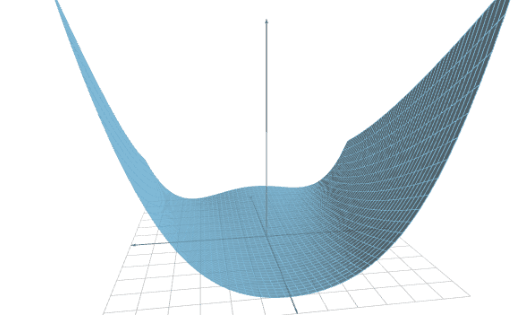﻿ The Rosenbrock benchmark suite for machine learning | Neural Designer

# The Rosenbrock Dataset Suite for benchmarking approximation algorithms and platforms

By Carlos Barranquero and Roberto Lopez, Artelnics.There are numerous repositories with a large number of datasets for machine learning. Some of the most important ones are the UCI Machine Learning Repository or Kaggle.

However, using that datasets for performance benchmarking might be difficult. Indeed, those datasets lack the consistency required by key performance indicators such as data capacity, training speed, model accuracy and inference speed.

This post introduces a family of datasets, known as the Rosenbrock Dataset Suite. The objective is to facilitate benchmarking of machine learning platforms.

Contents:

## Introduction### Data capacity tests

The data capacity of a machine learning platform can be defined as the biggest dataset that it is capable of processing. In this way, the tool should perform all the esential tasks with that dataset.

Data capacity can be measured as the number of samples that a machine learning platform can process, for a given number of variables.

The most important drawback is that they usually have a fixed number of variables and samples. This makes it difficult to test how a machine learning platform behaves with different dataset sizes.### Training speed tests

Training speed is defined as the number of samples per second that a machine learning platform processes during training.

The training speed depends very much on the dataset size. For instance, CPUs might provide faster training than GPUs for small datasets, and slower training for big datasets.

Therefore, we need to generate datasets with an arbitrary number of variables and samples, to see how these sizes affect training performance.### Model precision tests

Precision can be defined as the mean error of a model against a testing data set.

Most real datasets are noisy. This means that the full fit of the model to the data cannot be verified.

Therefore, it is desirable to have datasets with which we can potentially build models with zero error.### Inference speed tests

The inference speed can be defined as the time to calculate the outputs from the model as a function of the inputs. Inference speed is measured as the number of samples per second.

As before, we need to generate datasets with an arbitrary number of variables and samples, to see how these sizes affect training performance.

## Rosenbrock function

The Rosenbrock function is a non-convex function, introduced by Howard H. Rosenbrock in 1960. It is also known as Rosenbrock's valley or Rosenbrock's banana function.

It is used as a performance test problem for optimization algorithms.

In mathematical optimization, n is the number of samples and m is the number of input variables.

$$x_{i,j} = rand(-1,+1)$$ $$y_{j} = \sum_{i=1}^{n-1}\left[ 100\left(x_{i+1}-x_{i}^{2} \right)^{2}+\left(1-x_{i} \right)^{2} \right]$$

As the outputs from the Rosenbrock function are real values, this dataset suite is suitable for approximation problems. Therefore, we cannot test the performance of classification or forecasting applications with that.

The Rosenbrock dataset suite allows to create datasets with any number of variables and samples. Thus, this suite is perfect for performing data capacity, training speed and inference speed tests.

The Rosenbrock data is extracted from a deterministic function with a quite complex shape. It should be possible to build a machine learning model of that function with any desired degree of accuracy. Therefore, Rosenbrock datasets are ideal for model precision tests.

## C++ code

The following code shows how to generate a Rosenbrock dataset using C++.

// System includes

#include <iostream>
#include <fstream>
#include <string>
#include <random>

using namespace std;

int main(void)
{
cout << "Rosenbrock Dataset Generator." << endl;

const int inputs_number  = 2;
const int samples_number = 10000;

const string filename = "G:/R__" + to_string(samples_number)+ "_samples_"+ to_string(inputs_number) + "_inputs.csv";

float inputs[inputs_number];

default_random_engine generator;
uniform_real_distribution<float> distribution(-1.0, 1.0);

ofstream file(filename);

for(int j=0; j < samples_number; j++)
{
float rosenbrock = 0.0;

for(int i=0; i < inputs_number; i++)
{
inputs[i] = distribution(generator);

file << inputs[i] << ",";
}

for(int i = 0; i< inputs_number - 1; i++)
{
rosenbrock +=
(1 - inputs[i])*(1 - inputs[i])
+ 100*(inputs[i+1]-inputs[i] * inputs[i])*
(inputs[i+1]-inputs[i]*inputs[i]);
}

file << rosenbrock << endl;
}

file.close();

return 0;
}


## Python code

You can also generate a Rosenbrock dataset with the following Python code.

import numpy as np
import pandas as pd
import random

samples_number = 10000

inputs_number = 2

distribution = random.uniform(-1, 1)

inputs = np.random.uniform(-1.0, 1.0, size = (samples_number, inputs_number))

rosenbrock = []

for j in range (samples_number):

r = 0

for i in range(inputs_number-1):

r += (1.0 - inputs[j][i])*(1.0 - inputs[j][i])+100.0*((inputs[j][i+1]-inputs[j][i]*inputs[j][i])*(inputs[j][i+1]-inputs[j][i]*inputs[j][i]))

rosenbrock.append(r)

data = pd.concat([pd.DataFrame(inputs),pd.DataFrame(rosenbrock)], axis=1)

filename = "G:/R_" + str(samples_number)+ "_samples_"+ str(inputs_number) + "_variables_python.csv";

data.to_csv(filename,index = False,sep = ",")



Notice that data is normolized between [-1,1].

We provide the following datasets:

Rows\Columns 10 100 1000
1000 rosenbrok_103_10.csv rosenbrok_103_102.csv rosenbrok_103_103.csv
10000 rosenbrok_104_10.csv rosenbrok_104_102.csv rosenbrok_104_103.csv
100000 rosenbrok_105_10.csv rosenbrok_105_102.csv rosenbrok_105_103.csv
1000000 rosenbrok_106_10.csv rosenbrok_106_102.csv rosenbrok_106_103.csv

## Conclusions

This blog introduces a function to measure the data capacity, training speed, model accuracy, and inference speed for any machine learning platform. Rosenbrock datasets have a strong consistency and do not have noise. For this reason, it is a powerfull altenative to datasets from common repositories for benchmarking.

The data science and machine learning platform Neural Designer contains many utilities to perform descriptive, diagnostic, predictive, and prescriptive analytics easily.